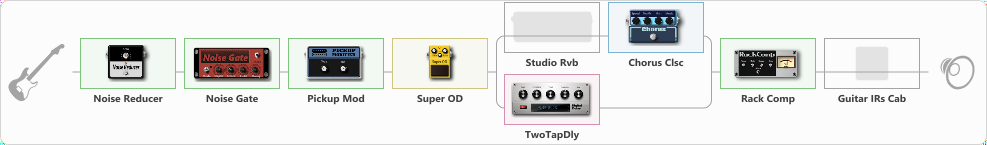# KINO - Kasparyan

Discussion in 'ToneLib-GFX presets' started by Ilnaz, Apr 30, 2023.

1. KINO - Kasparyan

Preset name: Каспарян

Effects chain:Effect: "Noise Reducer" (Dynamics / Filter), active - "yes"
{
"Sens" = 93
"Mode" = Hard
}

Effect: "Noise Gate" (Dynamics / Filter), active - "yes"
{
"Mode" = Manual
"Depth" = 0
"Threshold" = 54
"Attack" = 0
"Hold" = 5
"Decay" = 194
}

Effect: "Pickup Mod" (Dynamics / Filter), active - "yes"
{
"Mode" = Single->Humbacker
"Tone" = 40
}

Effect: "Super OD" (Overdrive / Distortion), active - "yes"
{
"Drive" = 67
"Tone" = 70
"Level" = 76
}

Effect: "Splitter" (Dynamics / Filter)
{
"A-Bypass" = Off
"A-Pan" = -25
"A-Level" = 55
"B-Bypass" = Off
"B-Pan" = 25
"B-Level" = 55
"Width" = 0

'A' branch:
{

Effect: "Studio Rvb" (Reverberation), active - "no"
{
"Time" = 7.4
"PreDelay" = 32
"LoDamp" = 0
"HiDamp" = 12
"Mix" = 58
}

Effect: "Chorus Clsc" (Modulation / Sfx), active - "yes"
{
"Speed" = 3.5
"Depth" = 58
"Center" = 1.0
"Mode" = Stereo
}
}
'B' branch:
{

Effect: "TwoTapDly" (Delay), active - "yes"
{
"Time" = 404
"Feedback" = 19
"Tone" = 65
"Sens" = 42
"TapTime" = 6.6
"Mix" = 68
}
}
}

Effect: "Rack Comp" (Dynamics / Filter), active - "yes"
{
"Threshold (dB)" = -14
"Ratio" = 2
"Attack" = Slow
"Release (ms)" = 250
"Knee" = 25
"Level (dB)" = 0
}

Effect: "Guitar IRs Cab" (Cabinets), active - "no"
{
"Model" = Marshall 1960A (4x12")
"Mic Position" = Middle
"Mic Distance" = Middle
"Low Cut (Hz)" = 60
"Hi Cut (kHz)" = 20.0
"Mix" = 69
"Level (dB)" = 3
}

Note: You will need to download and install the ToneLib-GFX software to use the preset.

File size:
939 bytes
Views:
789